A lot of people use the Google Sheets to do the calculation, for an example to calculate the revenue and profit. However, in the process of calculation, sometimes we will encounter the problem of the decimal point. And the different round off method will lead to a different calculation result. In this article, I am going to show you how to use the different round formulas includes round, rounddown, and roundup with Google Sheets Round Formula.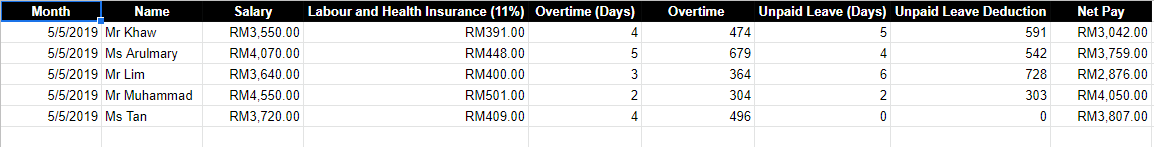In this article, I will take salary calculation as an example. It is because when calculating the employee salary, it is necessary to calculate the employee’s deductible expenses as well. The deductible expenses includes labor insurance and health insurance as well as the salary mobilization for leave or overtime. These figures are easy to encounter the situation of a decimal point, and it is easy to have errors if you use the wrong formula.

• ROUND Formula – Round a number according a standard rule
• ROUNDDOWN Formula – Round down a number
• ROUNDUP Formula – Round up a number

## ROUND Formula – Round a number according a standard rule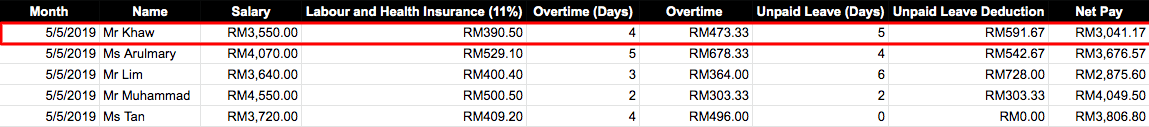Let’s calculate the first employee salary as shown in the picture above. If you calculate it before round off, the net pay for the first employee is RM 3,041.17.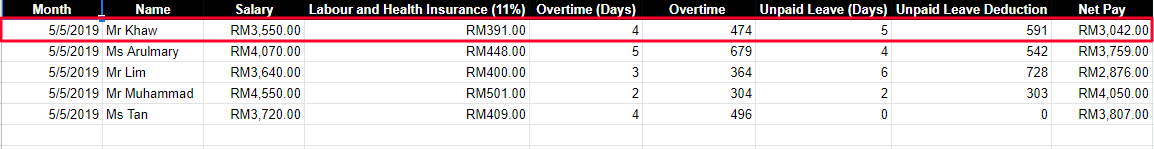However, if you are using round formula, you can get a more accurate number to meet your company rounding method. After rounding off, the salary become RM3042.

Let’s apply “ROUND”formula on the labor and health insurance as the image below: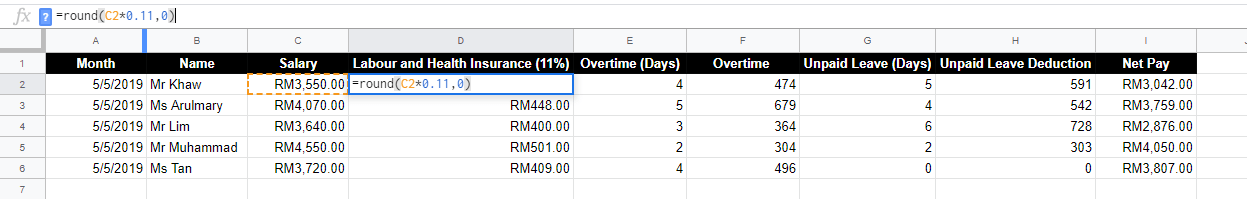ROUND(value,places), The 0 in this formula is rounded to the whole number, so if you want to go to the first decimal place you put a 1, if you want to go to the tenth place you put a -1, and so on

## ROUNDDOWN Formula – Round down a number

Assuming that your company stipulates that the salary deduction for leave is calculated to the integer bit by unconditional rounding. We use the formula ROUNDDOWN to calculate it. The formula is shown below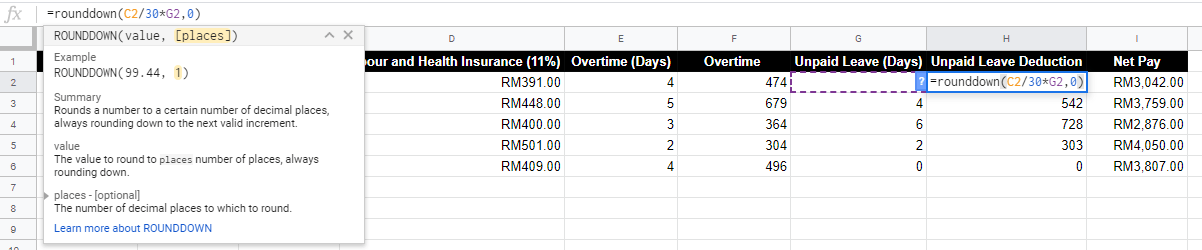ROUNDDOWN (value, pages), input is similar as the ROUND formula. The 0 in this formula is rounded to the whole number, so if you want to go to the first decimal place you put a 1. And if you want to go to the tenth place you put a -1, and so on

## ROUNDUP Formula – Round up a number

Lastly, I will cover the ROUNDUP formula. Assuming overtime working (OT) will be calculated to the integer bit by unconditional rounding. The formula is shown below.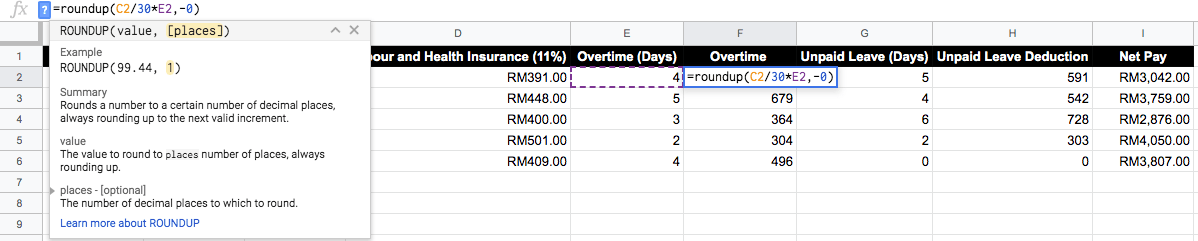ROUNDUP(values, pages),The 0 in this formula is rounded to the whole number. So, if you want to go to the first decimal place you put a 1. And if you want to go to the tenth place you put a -1, and so on.After we had applied these above ROUNDING formulas, you can see that we have the correct amount of Salary calculation. Do use the Google Sheets Round Formula to calculate numbers with decimal places.